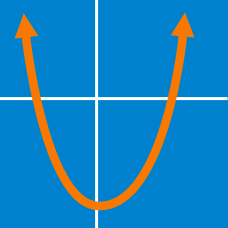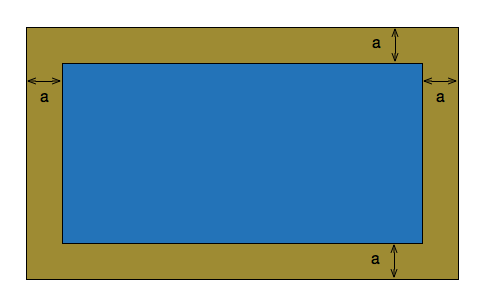Algebra

# Quadratic Equations - Problem Solving

If the roots of $ax^2+bx-2 =0$ are $\frac{1\pm\sqrt{3}}{3}$, what is a+b?

If the difference between the two roots of the equation $x^2-mx+5=0$ is $2,$ what is the value of $m^2?$

If the sum of the squares of three consecutive positive integers is $509,$ what is the sum of the three integers?In the above image, the blue picture is 3 inches longer than it is wide. On all sides, the yellow frame is 5 inches around. If the area of the picture is 378, what is the area of the frame?

What are the roots of the equation

$\frac{1}{x^2} + \frac{1}{ x} -20 = 0?$

×